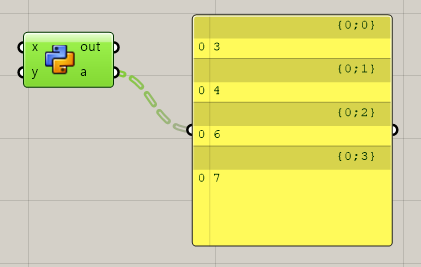# [Grasshopper] When creating a data tree on Python script

If you want to output in data tree format, you need to load the library and do some processing.

``````import System
import Grasshopper.Kernel.Data.GH_Path as GH_Path
import Grasshopper.DataTree as DataTree
``````

## ② Data tree output

This time, we will create a tree by extracting one data from the list data of [3, 4, 6, 7].

``````list = [3, 4, 6, 7]
tree = DataTree[System.Object]()
for i in range(len(list)):
path = GH_Path(0, i)
val = list[i]
It's easy once you remember it. It's a good idea to remember the above operations because you use them often. By the way, the output data tree is as follows.